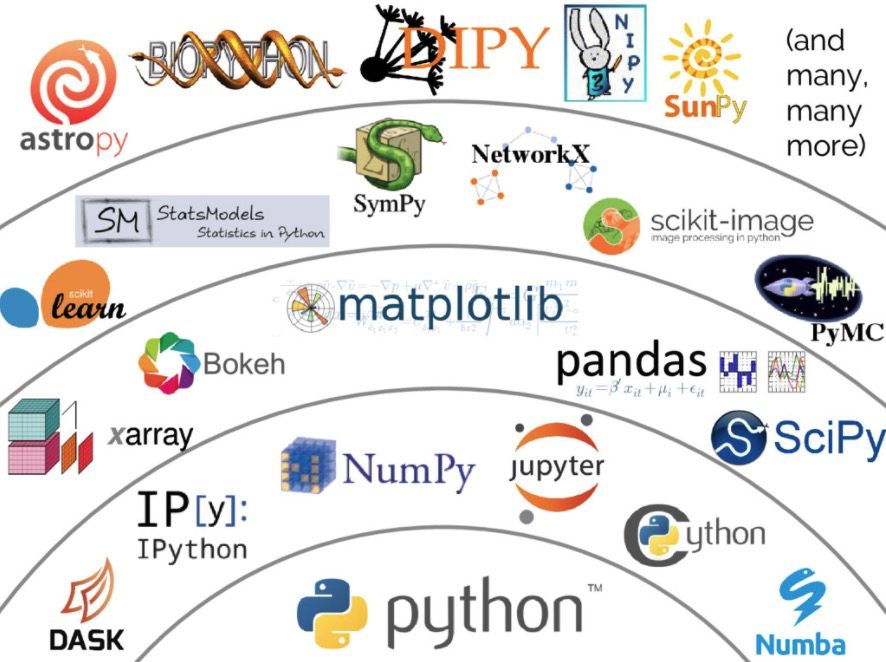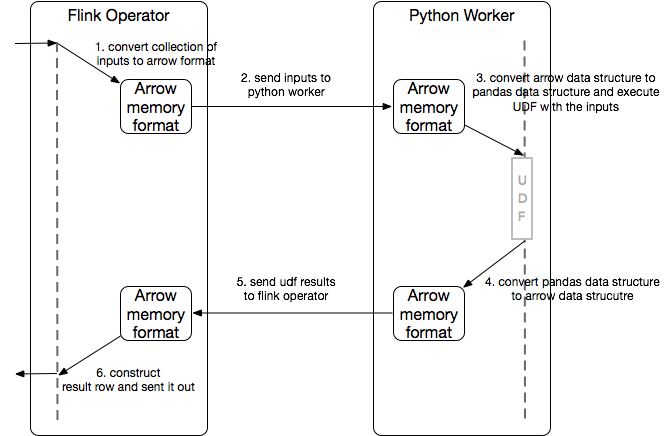04 Aug 2020 Jincheng Sun (@sunjincheng121) & Markos Sfikas (@MarkSfik)

Python has evolved into one of the most important programming languages for many fields of data processing. So big has been Python’s popularity, that it has pretty much become the default data processing language for data scientists. On top of that, there is a plethora of Python-based data processing tools such as NumPy, Pandas, and Scikit-learn that have gained additional popularity due to their flexibility or powerful functionalities.Pic source: VanderPlas 2017, slide 52.

In an effort to meet the user needs and demands, the Flink community hopes to leverage and make better use of these tools. Along this direction, the Flink community put some great effort in integrating Pandas into PyFlink with the latest Flink version 1.11. Some of the added features include support for Pandas UDF and the conversion between Pandas DataFrame and Table. Pandas UDF not only greatly improve the execution performance of Python UDF, but also make it more convenient for users to leverage libraries such as Pandas and NumPy in Python UDF. Additionally, providing support for the conversion between Pandas DataFrame and Table enables users to switch processing engines seamlessly without the need for an intermediate connector. In the remainder of this article, we will introduce how these functionalities work and how to use them with a step-by-step example.

Note Currently, only Scalar Pandas UDFs are supported in PyFlink.

# Pandas UDF in Flink 1.11

Using scalar Python UDF was already possible in Flink 1.10 as described in a previous article on the Flink blog. Scalar Python UDFs work based on three primary steps:

• the Java operator serializes one input row to bytes and sends them to the Python worker;

• the Python worker deserializes the input row and evaluates the Python UDF with it;

• the resulting row is serialized and sent back to the Java operator

While providing support for Python UDFs in PyFlink greatly improved the user experience, it had some drawbacks, namely resulting in:

• Difficulty when leveraging popular Python libraries used by data scientists — such as Pandas or NumPy — that provide high-performance data structure and functions.

The introduction of Pandas UDF is used to address these drawbacks. For Pandas UDF, a batch of rows is transferred between the JVM and PVM in a columnar format (Arrow memory format). The batch of rows will be converted into a collection of Pandas Series and will be transferred to the Pandas UDF to then leverage popular Python libraries (such as Pandas, or NumPy) for the Python UDF implementation.The performance of vectorized UDFs is usually much higher when compared to the normal Python UDF, as the serialization/deserialization overhead is minimized by falling back to Apache Arrow, while handling `pandas.Series` as input/output allows us to take full advantage of the Pandas and NumPy libraries, making it a popular solution to parallelize Machine Learning and other large-scale, distributed data science workloads (e.g. feature engineering, distributed model application).

# Conversion between PyFlink Table and Pandas DataFrame

Pandas DataFrame is the de-facto standard for working with tabular data in the Python community while PyFlink Table is Flink’s representation of the tabular data in Python. Enabling the conversion between PyFlink Table and Pandas DataFrame allows switching between PyFlink and Pandas seamlessly when processing data in Python. Users can process data by utilizing one execution engine and switch to a different one effortlessly. For example, in case users already have a Pandas DataFrame at hand and want to perform some expensive transformation, they can easily convert it to a PyFlink Table and leverage the power of the Flink engine. On the other hand, users can also convert a PyFlink Table to a Pandas DataFrame and perform the same transformation with the rich functionalities provided by the Pandas ecosystem.

# Examples

Using Python in Apache Flink requires installing PyFlink, which is available on PyPI and can be easily installed using `pip`. Before installing PyFlink, check the working version of Python running in your system using:

``````\$ python --version
Python 3.7.6``````

Note Please note that Python 3.5 or higher is required to install and run PyFlink

``\$ python -m pip install apache-flink``

## Using Pandas UDF

Pandas UDFs take `pandas.Series` as the input and return a `pandas.Series` of the same length as the output. Pandas UDFs can be used at the exact same place where non-Pandas functions are currently being utilized. To mark a UDF as a Pandas UDF, you only need to add an extra parameter udf_type=”pandas” in the udf decorator:

``````@udf(input_types=[DataTypes.STRING(), DataTypes.FLOAT()],
result_type=DataTypes.FLOAT(), udf_type='pandas')
def interpolate(id, temperature):
# takes id: pandas.Series and temperature: pandas.Series as input
df = pd.DataFrame({'id': id, 'temperature': temperature})

# use interpolate() to interpolate the missing temperature
interpolated_df = df.groupby('id').apply(
lambda group: group.interpolate(limit_direction='both'))

# output temperature: pandas.Series
return interpolated_df['temperature']``````

The Pandas UDF above uses the Pandas `dataframe.interpolate()` function to interpolate the missing temperature data for each equipment id. This is a common IoT scenario whereby each equipment/device reports it’s id and temperature to be analyzed, but the temperature field may be null due to various reasons. With the function, you can register and use it in the same way as the normal Python UDF. Below is a complete example of how to use the Pandas UDF in PyFlink.

``````from pyflink.datastream import StreamExecutionEnvironment
import pandas as pd

env = StreamExecutionEnvironment.get_execution_environment()
env.set_parallelism(1)
t_env = StreamTableEnvironment.create(env)
t_env.get_config().get_configuration().set_boolean("python.fn-execution.memory.managed", True)

@udf(input_types=[DataTypes.STRING(), DataTypes.FLOAT()],
result_type=DataTypes.FLOAT(), udf_type='pandas')
def interpolate(id, temperature):
# takes id: pandas.Series and temperature: pandas.Series as input
df = pd.DataFrame({'id': id, 'temperature': temperature})

# use interpolate() to interpolate the missing temperature
interpolated_df = df.groupby('id').apply(
lambda group: group.interpolate(limit_direction='both'))

# output temperature: pandas.Series
return interpolated_df['temperature']

t_env.register_function("interpolate", interpolate)

my_source_ddl = """
create table mySource (
id INT,
temperature FLOAT
) with (
'connector.type' = 'filesystem',
'format.type' = 'csv',
'connector.path' = '/tmp/input'
)
"""

my_sink_ddl = """
create table mySink (
id INT,
temperature FLOAT
) with (
'connector.type' = 'filesystem',
'format.type' = 'csv',
'connector.path' = '/tmp/output'
)
"""

t_env.execute_sql(my_source_ddl)
t_env.execute_sql(my_sink_ddl)

t_env.from_path('mySource')\
.select("id, interpolate(id, temperature) as temperature") \
.insert_into('mySink')

t_env.execute("pandas_udf_demo")``````

To submit the job:

• Firstly, you need to prepare the input data in the “/tmp/input” file. For example,
``\$ echo -e  "1,98.0\n1,\n1,100.0\n2,99.0" > /tmp/input``
• Next, you can run this example on the command line,
``\$ python pandas_udf_demo.py``

The command builds and runs the Python Table API program in a local mini-cluster. You can also submit the Python Table API program to a remote cluster using different command lines, see more details here.

• Finally, you can see the execution result on the command line. As you can see, all the temperature data with an empty value has been interpolated:
``````\$  cat /tmp/output
1,98.0
1,99.0
1,100.0
2,99.0``````

You can use the `from_pandas()` method to create a PyFlink Table from a Pandas DataFrame or use the `to_pandas()` method to convert a PyFlink Table to a Pandas DataFrame.

``````from pyflink.datastream import StreamExecutionEnvironment
import pandas as pd
import numpy as np

env = StreamExecutionEnvironment.get_execution_environment()
t_env = StreamTableEnvironment.create(env)

pdf = pd.DataFrame(np.random.rand(1000, 2))
table = t_env.from_pandas(pdf, ["a", "b"]).filter("a > 0.5")

# Convert the PyFlink Table to a Pandas DataFrame
pdf = table.to_pandas()
print(pdf)``````

# Conclusion & Upcoming work

In this article, we introduce the integration of Pandas in Flink 1.11, including Pandas UDF and the conversion between Table and Pandas. In fact, in the latest Apache Flink release, there are many excellent features added to PyFlink, such as support of User-defined Table functions and User-defined Metrics for Python UDFs. What’s more, from Flink 1.11, you can build PyFlink with Cython support and “Cythonize” your Python UDFs to substantially improve code execution speed (up to 30x faster, compared to Python UDFs in Flink 1.10).

Future work by the community will focus on adding more features and bringing additional optimizations with follow up releases. Such optimizations and additions include a Python DataStream API and more integration with the Python ecosystem, such as support for distributed Pandas in Flink. Stay tuned for more information and updates with the upcoming releases!